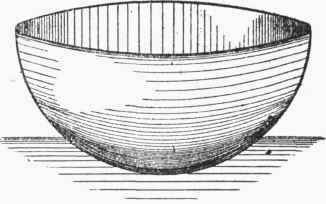The simplest article of hollow work to obtain the pattern for is probably that of a bowl as seen in Fig. 223. The size of disc for this can be obtained in several ways, all based upon the assumption that the area of the circular pattern is equal to the area of the curved surface of the bowl. The above assumption is practically correct, and would be strictly so if the metal of the hollowed bowl were exactly the same thickness as the sheet from which it had been worked up. To keep the metal the same thickness is almost impossible in practice; but what area is lost by contraction is generally balanced by that gained in expansion.

If the bowl is in shape a hemisphere, then the radius of the disc can be calculated if we remember that "the area of the surface of a complete sphere is equal to the square of the diameter multiplied by 3.1416." That is, area of sphere surface = d2 π, and as the area of the disc must be equal to half of the above, we have -

R2π = d2π / 2 .·. R2 = d2 / 2 and- R = d / √2 = 2 / 1.4 where R equals radius of pattern disc. This gives us a handy rule, for in all cases where the bowl is half a sphere, the radius of the circular pattern will be found by dividing the diameter of the sphere by 1.4.

For those who are not good at calculating the same result can be obtained graphically by aid of the construction shown in Fig. 224. This construction, indeed, may be taken generally and applied to all hollowed work that comes out as any segmental portion of a sphere. Essentially the construction consists in setting out a right-angle triangle, one side being equal to half the diameter of bowl, and the other equal to the depth; the hypotenuse or third side then giving the radius of the disc.Fig. 223.Fig. 224.

The general case is perhaps better explained by Fig. 225, the parallel lines representing the. top of bowls, and the dotted lines with the numbered R giving the radius of the corresponding required disc. Thus the line R1 will give the radius of a circular plate which will work up to a bowl whose top is represented by the line 1 1. In the same way a circular plate whose radius is equal to R3 would form a bowl whose diameter at top would be equal to 3 3.

From Fig. 225 it is interesting to observe that the radius of a circle having the same area as a sphere will be equal to the diameter of the sphere. So that, if it were possible to work a sheet metal disc into a complete ball of the sameFig. 225, thickness metal, the diameter of the disc would be just twice that of the sphere.

In calculating the surface area of a sphere, or any part of a spherical surface, it is often handy to call to mind the relation that exists between the surfaces of the sphere and cylinder. "The area of a sphere, or the curved surface of a segment or zone of a sphere, is equal to that of the circumscribing cylinder or part of cylinder." Thus, in Fig. 226, the area of the segment of sphere ABC will be equal to the area of the curved surface of the part of cylinder a c b b, which is cut off by A C, produced as shown. In the same way the area of the curved surface of zone will equal the part of cylinder cut away by producing D E and F G.

This somewhat peculiar property of the sphere and cylinder occasionally comes in handy in setting out patterns for plumber's and coppersmith's work, and also in estimating weights of sheet metal required in work of this character. It is also of use when the end of a straight pipe is required to be worked to form part of a dome end, such as in coppers, steam-dome covers, and coal-scoops. Referring again to Fig. 226, suppose we require to work the end of a straight pipe into the shape b H F, or b L G, then the length of pipe wanted to form the domical part H F would be H f. It should be borne in mind that if the point f is to be worked around to F it will be necessary in the raising to so stretch the metal as to keep it a constant thickness.Fig. 226.